# Data visualization index

These charts are developed and maintained by members of the IBM Carbon community. Some may only be accessible to IBMers. They may change over time, and they may be incomplete or experimental. For support, please contact the contributors listed on each page.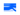Alluvial/sankey diagrams

Alluvial, or Sankey diagrams, are a type flow diagram designed to show two indicators of a dataset and how records distribute among them, highlighting correlations.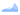Area chart

Area charts are similar to line charts, but the areas below the lines are filled with colors or patterns. Stacked charts are useful for comparing proportional contributions within a category.Area chart

Area charts are similar to line charts, but the areas below the lines are filled with colors or patterns. Stacked charts are useful for comparing proportional contributions within a category.Bar chart

Bar charts use horizontal rectangular bars to answer the question of "how many?" in each category.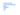Bar horizontal chart

Bar charts use vertical or horizontal data markers to compare individual values. You can use them to compare discrete data or show trends over time.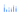Bar vertical chart

Bar charts use vertical or horizontal data markers to compare individual values. You can use them to compare discrete data or show trends over time.Boxplot

A box and whisker plot (or box plot) is a convenient way of visually displaying the data distribution through their quartiles.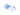Bubble chart

Bubble charts use data points and bubbles to plot measures anywhere along a scale. One measure is plotted along each axis. The size of the bubble represents the third measure.Bubble chart

A bubble chart is similar to a scatter plot in that it can show distribution or relationship. The third data set is indicated by the size of the bubble or circle.Bubblemap

A bubble map chart is simply a combination of a bubble chart data visualization and a map. It is used to visualize location and proportion in a simple way.Bullet graph

A bullet graph reveals progress toward a goal, compares this to another measure, and provides context in the form of a rating or performance.Chloropeth

A choropleth map is a thematic map in which areas are shaded or patterned in proportion to the measurement of the statistical variable being displayed on the map, such as population density or per-capita income.Circle pack

Circle packing is a method to visualize large amounts of hierarchically structured data and is a variation of a tree map that uses circles instead of rectangles.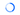Donut chart

Donut chart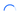Gauge chart

Gauge chart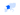Geospatial charts

Geospatial charts are a collection of chart types that overlay over geographical data.Heat map

A heat map is a two-dimensional visualization in which individual values contained in a matrix are represented as colors. This technique makes it easy to visualize complex data at a glance.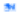Heat maps

A heat map is a two-dimensional visualization in which individual values contained in a matrix are represented as colors. This technique makes it easy to visualize complex data at a glance.Histogram

A histogram visualizes the distribution of data over a continuous interval or certain time period.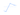Line chart

Line charts plot data at regular intervals connected by lines. You can use line visualizations to show trends over time and compare several data sets.Line graph

Use a line graph to reveal trends or progress over time with a continuous data set. A line graph is created by connecting a series of data points together with a line.Master detail

Create a master detail relationship to deliver information that would otherwise require two or more reports. For example, you can combine a list with a chart. The list can contain product lines and the chart can show details for each product line.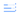Meter chart

Scatter plot visualizations use data points to plot two measures anywhere along a scale, not only at regular tick marks. You can use scatter plots to explore correlations between different measures.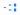Network diagrams

A network diagram is a way of visually representing network architecture. It maps out network structure with a variety of icons and connecting lines.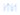Parallel coordinates

Parallel coordinate charts visualize individual data elements across multiple variables. Each of variable corresponds to a vertical axis and each data element is displayed as a series of connected points along the axes.Pie / donut / gauge charts

Use pie charts and donut charts very sparingly, and only to show a part-to-a-whole relationship. Pie charts and donut charts show how categories represent part of a whole – the composition of something.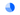Pie chart

Pie chart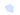Scatter plot visualizations use data points to plot two measures anywhere along a scale, not only at regular tick marks. You can use scatter plots to explore correlations between different measures.Sankey diagram

Sankey diagrams display flows and their quantities in proportion to one another. The width of the arrows or lines are used to show their magnitudes, so the bigger the arrow, the larger the quantity of flow.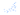Scatter plot

Scatter plot visualizations use data points to plot two measures anywhere along a scale, not only at regular tick marks. You can use scatter plots to explore correlations between different measures.Scatter plot

A scatter plot, or scattergram chart, will show the relationship between two different variables or it can reveal the distribution trends.Step chart

Stepped line charts plot data at regular intervals, forming a series of steps between data points. You can use line visualizations to show trends over time and compare several data sets.Stream graph

A stream graph is a variation of a stacked area chart. Instead of plotting values against a fixed, straight axis, a stream graph has values displaced around a varying central baseline, resulting in a flowing, organic shape.Sunburst diagram

A sunburst diagram is used to visualize hierarchical data, depicted by concentric circles that are sliced for each category node.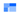Tree maps

Tree maps can work for visualizing a part-to-whole relationship among a large number of categories—as long as the data is hierarchical and exact comparisons between the categories is not a primary concern.Treemap graph

Tree maps are an alternative way of visualizing the hierarchical structure of a tree diagram while also displaying quantities for each category via area size.Violin plot

A violin plot is a method of plotting numeric data. It is a combination of a box plot and a density plot that is rotated and placed on each side, to show the distribution shape of the data.Weighted tree

The weighted tree is an ideal way to represent asymmetric hierarchal information in an easy to read visualization.

Filters
Maintainer
Chart type
Complexity
Availability